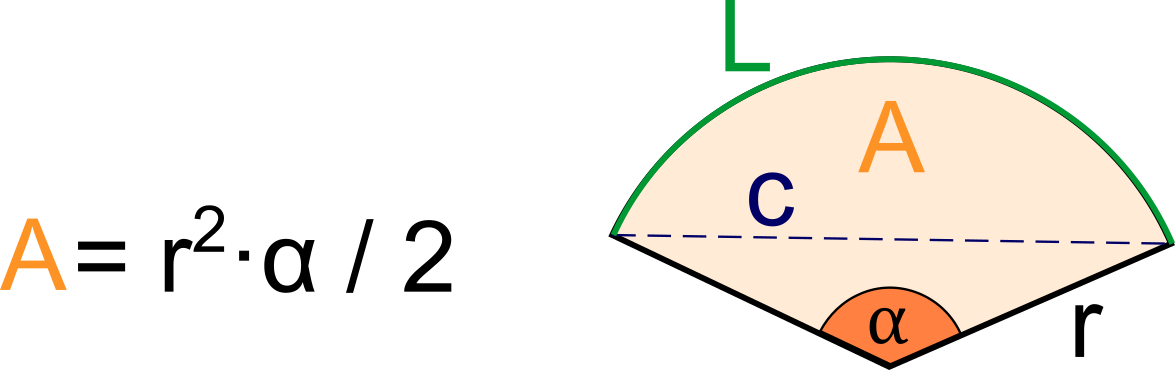# Sector Area Calculator

Created by Hanna Pamuła, PhD candidate
Reviewed by Bogna Szyk and Jack Bowater
Last updated: Aug 27, 2022

With this sector area calculator, you'll quickly find any circle sector area, e.g., the area of semicircle or quadrant. In this short article we'll:

• provide a sector definition and explain what a sector of a circle is.
• show the sector area formula and explain how to derive the equation yourself without much effort.
• reveal some real-life examples where the sector area calculator may come in handy.

## What is a sector of a circle? Sector definition

So let's start with the sector definition - what is a sector in geometry?

A sector is a geometric figure bounded by two radii and the included arc of a circle

Sectors of a circle are most commonly visualized in pie charts, where a circle is divided into several sectors to show the weightage of each segment. The pictures below show a few examples of circle sectors - it doesn't necessarily mean that they will look like a pie slice, sometimes it looks like the rest of the pie after you've taken a slice:You may, very rarely, hear about the sector of an ellipse, but the formulas are way, way more difficult to use than the circle sector area equations.

## Sector area formula

The formula for sector area is simple - multiply the central angle by the radius squared, and divide by 2:

• Sector Area = r² × α / 2

But where does it come from? You can find it by using proportions, all you need to remember is circle area formula (and we bet you do!):

1. The area of a circle is calculated as A = πr². This is a great starting point.
2. The full angle is 2π in radians, or 360° in degrees, the latter of which is the more common angle unit.
3. Then, we want to calculate the area of a part of a circle, expressed by the central angle.
• For angles of 2π (full circle), the area is equal to πr²: 2π → πr²
• So, what's the area for the sector of a circle: α → Sector Area
1. From the proportion we can easily find the final sector area formula:

Sector Area = α × πr² / 2π = α × r² / 2

The same method may be used to find arc length - all you need to remember is the formula for a circle's circumference.

💡 Note that α should be in radians when using the given formula. If you know your sector's central angle in degrees, multiply it first by π/180° to find its equivalent value in radians. Or you can use this formula instead, where θ is the central angle in degrees:

Sector Area = r² × θ × π / 360

## Special cases: area of semicircle, area of quadrant

Finding the area of a semicircle or quadrant should be a piece of cake now, just think about what part of a circle they are!

1. Semicircle area: πr² / 2• Knowing that it's half of the circle, divide the area by 2:

Semicircle area = Circle area / 2 = πr² / 2

• Of course, you'll get the same result when using sector area formula. Just remember that straight angle is π (180°):

Semicircle area = α × r² / 2 = πr² / 2

1. Quadrant area: πr² / 4• As quadrant is a quarter of a circle, we can write the formula as:

Quadrant area = Circle area / 4 = πr² / 4

• Quadrant's central angle is a right angle (π/2 or 90°), so you'll quickly come to the same equation:

Quadrant area = α × r² / 2 = πr² / 4

## Sector area calculator – when it may be useful?

We know, we know: "why do we need to learn that, we're never ever gonna use it". Well, we'd like to show you that geometry is all around us:

• If you're wondering how big cake you should order for your awesome birthday party - bingo, that's it! Use sector area formula to estimate the size of a slice 🍰 for your guests so that nobody will starve to death. Check out how we've implemented it in our cake serving calculator.
• It's a similar story with pizza - have you noticed that every slice is a sector of a circle 🍕? For example, if you're not a big fan of the crust, you can calculate which pizza size will give you the best deal (don't forget about the tip afterwards).
• Any sewing enthusiasts here?👗 Sector area calculations may be useful in preparing a circle skirt (as it's not always a full circle but, you know, a sector of a circle instead).

Apart those simple, real-life examples, the sector area formula may be handy in geometry, e.g. for finding surface area of a cone.

Hanna Pamuła, PhD candidate
Central angle
deg
in
Diameter
inSector area (A)
in²
Arc length (L)
in
Chord length (c)
in
People also viewed…

### Cubic yards in a cylinder

Our cubic yards in a cylinder calculator will help you to calculate the volume of the cylinder in cubic yards.

### Integer

The integer calculator is here to calculate all your math operations involving integers (including negative numbers).

### Korean age

If you're wondering what would your age be from a Korean perspective, use this Korean age calculator to find out.

### Steps to calories

Steps to calories calculator helps you to estimate the total amount to calories burned while walking.